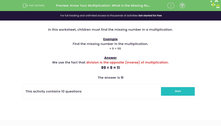# Use Division as the Inverse Operation of Multiplication

In this worksheet, students will use division to work out the missing number in a multiplication.Key stage:  KS 2

Curriculum topic:   Number: Multiplication and Division

Curriculum subtopic:   Use Multiplication/Division Facts

Popular topics:   Multiplication worksheets

Difficulty level:#### Worksheet Overview

In this activity, we will be finding the missing number in a multiplication.

Example

Find the missing number in the multiplication.

× 9 = 99

We use the fact that division is the opposite (inverse) of multiplication.

99 ÷ 9 = 11

We can always check that we're right by putting our answer into the original sentence: 11 x 9 = 99

We're correct!Let's try some questions now!

### What is EdPlace?

We're your National Curriculum aligned online education content provider helping each child succeed in English, maths and science from year 1 to GCSE. With an EdPlace account you’ll be able to track and measure progress, helping each child achieve their best. We build confidence and attainment by personalising each child’s learning at a level that suits them.

Get started